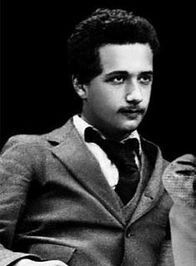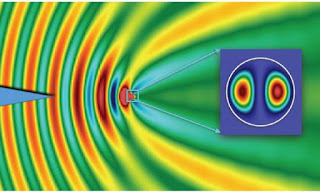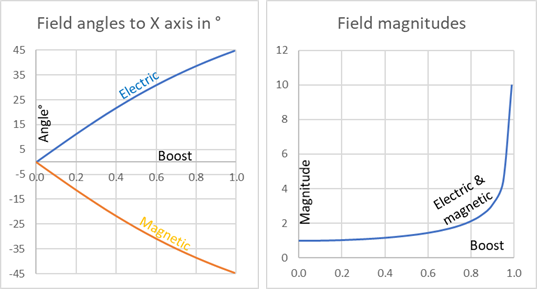## Tuesday 25 February 2020

### Einstein's equationEinstein age 18. Credit.
We are now on section 4.2 which has some very shady approximations. However we do get to Einstein's equation for general relativity if we tolerate that. The equation is$$R_{\mu\nu}-\frac{1}{2}Rg_{\mu\nu}=8\pi GT_{\mu\nu}$$where $R_{\mu\nu},R$ are the Ricci tensor and scalar which tell us about the curvature of spacetime, $g_{\mu\nu}$ is the metric, $G$ is Newton's constant and $T_{\mu\nu}$ is the energy-momentum tensor. So the equation tells us how the curvature of spacetime reacts to the presence of energy-momentum (which includes mass). Newton is not forgotten altogether😊.

The equation can also be written as $$R_{\mu\nu}=8\pi G\left(T_{\mu\nu}-\frac{1}{2}Tg_{\mu\nu}\right)$$where $T=g_{\mu\nu}T_{\mu\nu}$ and in empty space where $T_{\mu\nu}=0$ that gives us$$R_{\mu\nu}=0$$The equation is a field equation for the metric and the Newtonian gravity field equation is Poisson's equation$$\nabla^2\Phi=4\pi G\rho$$where $\Phi$ is the gravitational potential and $\rho$ the mass density.

The section starts by plausibly guessing that GR field equation must be of the form $$R_{\mu\nu}-\frac{1}{2}Rg_{\mu\nu}=\kappa T_{\mu\nu}$$where $\kappa$ is a constant we must find. The GR field equation must be the same as Poisson's equation in almost-flat spacetime. So we use a small perturbation $h_{\mu\nu}$ on the flat metric: $$g_{\mu\nu}=\eta_{\mu\nu}+h_{\mu\nu}$$and discarding second order terms in $h_{\mu\nu}$ eventually work out that $\kappa=8\pi G$ to bring the two equations into line.

However! Carroll's 4.38 is wrong. It says $T_{00}=\rho$ and in fact $T_{00}=\rho\left(1-h_{00}\right)$ and  if 4.38 were right then 4.39 would be wrong, but in fact it is right. Carroll is sort of having it both ways and we only get $\ \kappa\approx8\pi G$ at best. It contains first order terms in $h_00$. Hopefully the next section using the Lagrangian formulation will do better!

See Commentary 4.2 Einsteins equation.pdf (5 pages) for the details.

## Sunday 23 February 2020

### Our local star

Thanks to Scientific American. The original on their site looks better!

## Thursday 20 February 2020

### Exercise 1.12 Energy momentum tensors of two field theoriesEM waves by Lomonosov Moscow State University
Question
Consider the two field theories we explicitly discussed, Maxwell's electromagnetism (let $J^\mu=0$) and the scalar field theory defined by (1.148)

(a) Express the components of the energy-momentum tensors of each theory in three-vector notation, using divergence, gradient, curl, electric and magnetic fields, and an overdot to denote time derivatives.

(b) Using the equations of motion, verify (in any notation you like) that the energy-momentum tensors are conserved.

The energy momentum tensors in question were
\begin{align}
T_{scalar}^{\mu\nu}=\eta^{\mu\lambda}\eta^{\nu\sigma}\partial_\lambda\phi\partial_\sigma\phi-\eta^{\mu\nu}\left(\frac{1}{2}\eta^{\lambda\sigma}\partial_\lambda\phi\partial_\sigma\phi+V\left(\phi\right)\right)&\phantom {10000}(1)\nonumber
\end{align}and
\begin{align}
T_{EM}^{\mu\nu}=F^{\mu\lambda}F_{\ \ \ \lambda}^\nu-\frac{1}{4}\eta^{\mu\nu}F^{\lambda\sigma}F_{\lambda\sigma}&\phantom {10000}(2)\nonumber
We had to answer a and b for each tensor so that makes four questions really.

a scalar) The first one was quite easy but we had to use the outer product $\bigotimes$ and the Kronecker delta $\delta^{ij}$ too. (Assuming $\nabla\phi\bigotimes\nabla\phi$ means what I think it means.)

a TM) The second was quite difficult and I found a new tool Microsoft Mathematics to multiply horrid matrices which come from the likes of $F^{\mu\lambda}F_{\ \ \ \lambda}^\nu$ in (2).

In both the b cases we need to show that the tensors are conserved we just have to show that
\begin{align}
\partial_\mu T^{\mu\nu}=0&\phantom {10000}(3)\nonumber
\end{align}which is four equations one for each $\nu$, the four coordinates.

b scalar) I was able to do that entirely by tensor and index manipulation which was cool. The time and space coordinates worked the same.

b EM) This was a horrible mixture of tensor and index manipulation and then decomposition into electric and magnetic fields. I had to do the $t,x$ coordinates separately and assumed that  $y,z$ are like $x$. It was nasty.

Read the full answer here: Ex 1.12 Energy momentum tensors of two field theories.pdf

## Wednesday 19 February 2020

### Microsoft Mathematics

I was recently doing a problem which involved calculating the electromagnetic energy momentum tensor$$T^{\mu\nu}=F^{\mu\lambda}F_{\ \ \ \lambda}^\nu-\frac{1}{4}\eta^{\mu\nu}F^{\lambda\sigma}F_{\lambda\sigma}$$In a previous problem I had already calculate$$F_{\mu\nu}=\left[\begin{matrix}0&-E_1&-E_2&-E_3\\E_1&0&B_3&-B_2\\E_2&-B_3&0&B_1\\E_3&B_2&-B_1&0\\\end{matrix}\right],\ F_{\ \ \ \lambda}^\nu=\eta^{\nu\rho}F_{\rho\lambda}=\left[\begin{matrix}0&E_1&E_2&E_3\\E_1&0&B_3&-B_2\\E_2&-B_3&0&B_1\\E_3&B_2&-B_1&0\\\end{matrix}\right]$$
and$$F^{\mu\nu}=F_{\ \ \ \lambda}^\mu\eta^{\lambda\nu}=\left[\begin{matrix}0&E_1&E_2&E_3\\-E_1&0&B_3&-B_2\\-E_2&-B_3&0&B_1\\{-E}_3&B_2&-B_1&0\\\end{matrix}\right]$$I was using $c=1$ and metric signature $-++++$
So I had to do a bit of unpleasant matrix multiplication. I found Microsoft Mathematics useful. It successfully tells me that $$F^{\mu\lambda}F_{\ \ \ \lambda}^\nu=\left[\begin{matrix}{E_1}^2+{E_2}^2+{E_3}^2&B_3E_2-B_2E_3&B_1E_3-B_3E_1&B_2E_1-B_1E_2\\B_3E_2-B_2E_3&{B_2}^2+{B_3}^2-{E_1}^2&-B_1B_2-E_1E_2&-B_1B_3-E_1E_3\\B_1E_3-B_3E_1&-B_1B_2-E_1E_2&{B_1}^2+{B_3}^2-{E_2}^2&-B_2B_3-E_2E_3\\B_2E_1-B_1E_2&-B_1B_3-E_1E_3&-B_2B_3-E_2E_3&{B_1}^2+{B_2}^2-{E_3}^2\\\end{matrix}\right]$$
(without working out that ${E_1}^2+{E_2}^2+{E_3}^2=E$) and was good at the other bits. The above matrix will easily paste back into a MS-Word equation. Sadly one cannot paste matrices from Word into it.

Subscripted variables can easily be entered as E_x becomes $E_x$. Greek letters are allowed too. Superscripts are always exponents. It also does differentiation, integration and quite a bit more which I have not explored.

It has some drawbacks on my computer at least.

As mentioned I was unable to copy/paste matrices from MS-Equations into MS-Mathematics, so all the data entry must be done in the latter. There is an addin which might do the trick. But it did not like my version of Word (Office 365). The main install program, crashed when it came to the addin part.

The 'keyboard' is tiny and the seven choices of colours are demented. The image on the right is about the right size but it is less legible than the real thing.

## Sunday 16 February 2020

### Classical field theorySir William Rowan Hamilton
I really want to do section 4.3 the Lagrangian formulation of general relativity but first I am going back to section 1.10 on classical field theory which I skipped  because it involved the mysterious Lagrangian. I also needed to know about the fascinating Taylor expansions (Commentary 1.10 Taylor and Maclaurin series) and its extension for a function of two variables (which I guessed to begin with). My investigations on the Laplace operator or Laplacian ($\nabla^2$ see Commentary 4.1 Laplacian) also came in handy.

Considering the number of things I did not know it was a good idea to postpone reading this section until now.

The section describes how we use Hamilton's least action principle and the Lagrangian which becomes the Lagrange density to get field equations.
It then has examples of how to use the procedure to get
• a scalar field theory,
• a field theory for a harmonic oscillator which might be related to the relativistic equation of motion of an electron and
• an Electro Magnetic field theory which (with some assumptions) gives Maxwell's equations.
The key thing is to guess the Lagrange density in each case .

Read about my chapter 1 "Endkampf" here: Commentary 1.10 Classical Field Theory.pdf (9 pages)

## Question

Using the tensor transformation law applied to $F_{\mu\nu}$, show how the electric magnetic field 3-vectors $E$ and $B$ transform under
a) a rotation about the $y$-axis,
b) a boost along the $z$-axis.Electric and magnetic fields increase and contra-rotate perpendicular to direction of relative motion. Boost is relative speed in $z$-direction as a fraction of $c$ speed of light.
Carroll has already given us the Electro Magnetic Field Tensor is $$F_{\mu\nu}=\left[\begin{matrix}0&-E_1&-E_2&-E_3\\E_1&0&B_3&-B_2\\E_2&-B_3&0&B_1\\E_3&B_2&-B_1&0\\\end{matrix}\right]=-F_{\nu\mu}$$and the Lorentz rotation transformation matrix $$\Lambda_{\ \ \nu}^{\mu^\prime}=\left[\begin{matrix}1&0&0&0\\0&\cos{\theta}&0&\sin{\theta}\\0&0&1&0\\0&-\sin{\theta}&0&\cos{\theta}\\\end{matrix}\right]$$and the Lorentz transformation under a boost $v$ along the $z$-axis $$\Lambda_{\ \ \mu}^{\mu^\prime}=\left[\begin{matrix}\cosh{\phi}&0&0&-\sinh{\phi}\\0&1&0&0\\0&0&1&0\\-\sinh{\phi}&0&0&\cosh{\phi}\\\end{matrix}\right]$$Where the boost parameter $\phi=\tanh^{-1}{v}$.

There are many ways to solve this problem and I learnt many lessons.

### Method 1The Cartesian rotation matrix by geometry.
The simplest way to do the first question (a) is just to use the Cartesian rotation matrix on $E$ and $B$. For any vector $X$ that gives $$\left(\begin{matrix}{X^\prime}_1\\{X^\prime}_2\\{X^\prime}_3\\\end{matrix}\right)=\left(\begin{matrix}X_1\cos{\theta}+X_3\sin{\theta}\\X_2\\-X_1\sin{\theta}+X_3\cos{\theta}\\\end{matrix}\right)$$Put $E$ then $B$ into that and back into the equation for $F_{\mu\nu}$ and you get the answer. But that would be cheating and will not work for (b). On the other hand, it is useful to check the answer.

### Method 2

I found a crib-sheet that helped me here: One really should apply $\Lambda$ to $F$ but as it stands $\Lambda_{\ \ \nu}^{\mu^\prime}$ will only work on $F^{\mu\nu}$  not on $F_{\mu\nu}$. So one needs to get $\Lambda_{\mu^\prime}^{\ \ \ \ \nu}$ to operate on$\ F_{\mu\nu}$ or $F^{\mu\nu}$ to be operated on by $\Lambda_{\ \ \nu}^{\mu^\prime}$. We have$$\ F^{\mu\nu}=\eta^{\nu\sigma}\eta^{\mu\rho}F_{\rho\sigma}$$then we would get$$F^{\mu^\prime\nu^\prime}=\Lambda_{\ \ \mu}^{\mu^\prime}\Lambda_{\ \ \nu}^{\nu^\prime}F^{\mu\nu}$$and one can find the transformed $E,B$ by comparing $F^{\mu^\prime\nu^\prime}$ and $F^{\mu\nu}$.
The crib-sheet recommended to just do the sums that are implicit in the repeated $\mu,\nu$  indices in second equation. It is definitely easier to do two separate matrix multiplications! One has to be careful with where the indices are. I learnt about that as well.

### Method 3

Just before I finished I proved that the transformation $\ \Lambda_{\mu^\prime}^{\ \ \ \ \nu}$ to operate on the covariant $F_{\mu\nu}$ is simply the inverse of $\Lambda_{\ \ \nu}^{\mu^\prime}$ the original, useless, transformation. The inverse of $\Lambda_{\ \ \nu}^{\mu^\prime}$ can be found by making  $\theta\rightarrow-\theta$ or $\phi\rightarrow-\phi$ - reversing the angle or boost. So the answer can be obtained with many fewer calculations. Duh!

Full answer at Ex 1.10 Transformations of Electro Magnetic Field Tensor.pdf (11 pages!)

## Monday 3 February 2020

### Taylor and Maclaurin series

In section 1.10 Carroll Taylor-expands a Lagrangian. So I had a look at Taylor series. There is a very good article on Wikipedia about them.

In particular I looked at the Taylor series of 1/(1-x), polynomials especially 3rd order, sines and 'other functions' which states that they often converge to their Taylor series expansion which is a technique often used in physics.

I was able to proved that a 3rd order polynomial is its Taylor series, that is:$$k_0+k_1x+k_2x^2+k_3x^3=\sum_{i=0}^{i=3}\left(\left(\sum_{n=i}^{n=3}{\frac{n!}{\left(n-i\right)!}k_na^{n-i}}\right)\left(\sum_{j=0}^{j=i}\frac{x^{i-j}\left(-a\right)^j}{j!\left(i-j\right)!}\right)\right)$$The bit on the right is the Taylor series (after a bit of work) and there are many terms like  $a^ix^j$ which amazingly cancel each other out. I was not able to prove that any polynomial is its Taylor series as Wikipedia states.

The Taylor series for the sine function is $$x-\frac{x^3}{3!}+\frac{x^5}{5!}-\frac{x^7}{7!}+\ldots$$which is Madhava's sine series, the well known formula for $\sin{x}$. It was discovered in the west by Isaac Newton (1670) and Wilhelm Leibniz (1676). Taylor prospered in the 1700's so perhaps he found it independently. But since Newton and Leibniz independently invented calculus and argued about who was first, they probably new quite a bit about Taylor and McLaurin series. However they were all preceded by Madhava of Sangamagrama (c. 1350 – c. 1425), the founder of the Kerala school of astronomy and mathematics, who proved it as recorded in 1530 in the Indian text the Yuktibhāṣā. The picture shows the sine Taylor series for terms up to ${x^15}/{15!}$. Amazingly accurate!
Read it all in Commentary 1.10 Taylor and Maclaurin series.pdf (4 pages)